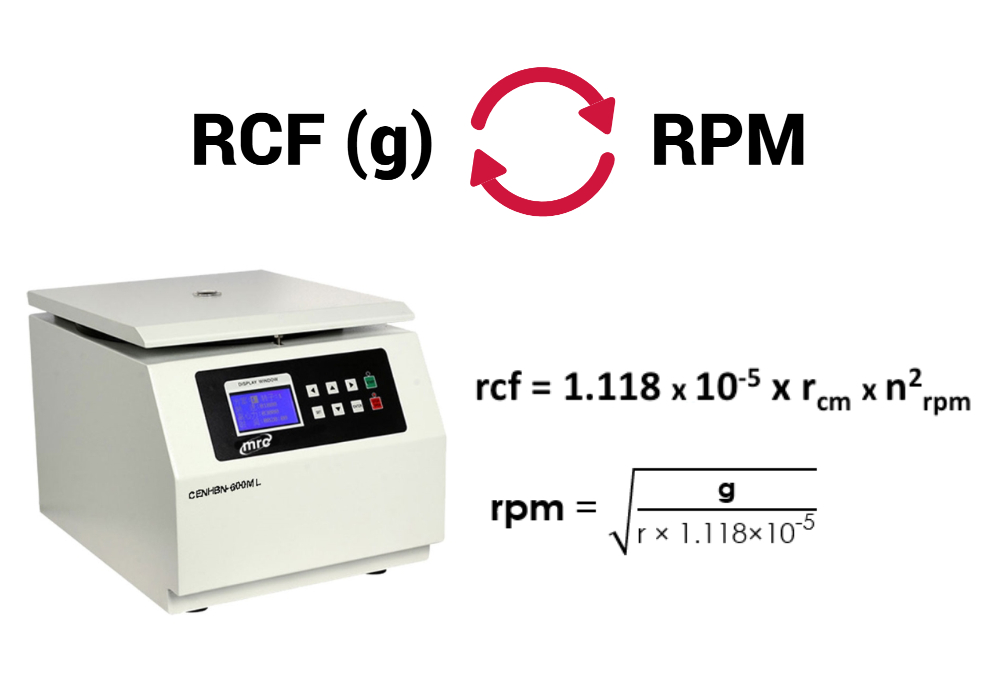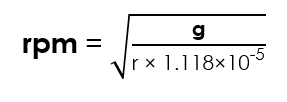# How to Convert ‘g’ to RPM and Vice Versa in a Centrifuge?Centrifuagtion is a technique that helps to separate mixtures by applying centrifugal force. A centrifuge machine works by using a principle of sedimentation. Under the influence of gravitational force, substances separate according to their density.

When dealing with centrifuge machines, we come across two different units of measurement: The Revolutions per minute (RPM) and Relative centrifugal force (RCF) or g-force. In fact these units are not same. This article discusses about what they actually are and how they are realated to each other.

## Revolutions per minute (RPM)

RPM (Revolutions per minute) basically describes how fast the centrifuge goes. It is a measurement of how fast the centrifuge rotor does a full rotation in one minute. The force applied to the contents varies by the size of the centrifuge rotor.

## Relative centrifugal force (RCF)

RCF (Relative centrifugal force) is the amount of force exerted on the contents on the rotor, resulting from revolutions of the rotor. It depends on the rotation speed and radius of the rotor. It is relative to the force of earth’s gravity and is measured in force*gravity (or g-force).

## Which one to use?

The bigger the radius the more acceleration is applied to the samples for the same RPM. Since different centrifuge machines may have different rotor sizes, using RCF or g-force will give very accurate setting for experiments. RCF remains constant irrelevant of the centrifuge you are using

## Converting RPM to RCF and vice versa

To calculate RCF from RPM, use following equation:To calculate RPM from RCF, use following equation:Where,

• RCF(g) = Relative Centrifugal Force
• r = Radius of the rotor (cm). It is the distance from the rotor axis to the bottom of the tube.
• RPM(n) = Revolutions Per Minute

## References

1. https://handling-solutions.eppendorf.com/sample-handling/centrifugation/safe-use-of-centrifuges/basics-in-centrifugation/
2. https://www.westlab.com/blog/2019/01/29/difference-between-rcf-and-rpm-in-centrifugation
3. https://blog.btlabsystems.com/blog/rpm-rcf-g-force
4. http://www.fao.org/3/ac802e/ac802e0u.htm
1.ABDULLAHI MUHAMMAD says: